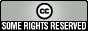Improving economics teaching and learning for over 20 years

# What do we mean by "statistics"?

Ron Smith, Birkbeck, University of London
Put online February 2010

This text is extracted from Applied Statistics and Econometrics: Notes and Exercises, one of the course documents shared through the TRUE wiki for Econometrics. It is available under a Creative Commons license, some rights reserved.

The word statistics has at least three meanings. Firstly, it is the data themselves, e.g. the numbers that the Office of National Statistics collects. Secondly, it has a technical meaning as measures calculated from the data, e.g. an average. Thirdly, it is the academic subject which studies how we make inferences from the data.

Descriptive statistics provide informative summaries (e.g. averages) or presentations (e.g. graphs) of the data. We will consider this type of statistics first.

Whether a particular summary of the data is useful or not depends on what you want it for. You will have to judge the quality of the summary in terms of the purpose for it is used. Different summaries are useful for different purposes.

Statistical inference starts from an explicit probability model of how the data were generated. For instance, an empirical demand curve says quantity demanded depends on income, price and random factors, which we model using probability theory. The model often involves some unknown parameters, such as the price elasticity of demand for a product. We then ask how to get an estimate of this unknown parameter from a sample of observations on price charged and quantity sold of this product. There are usually lots of different ways to estimate the parameter and thus lots of different estimators: rules for calculating an estimate from the data. Some ways will tend to give good estimates, some bad, so we need to study the properties of different estimators. Whether a particular estimator is good or bad depends on the purpose.

For instance, there are three common measures (estimators) of the typical value (central tendency) of a set of observations: the arithmetic mean or average; the median, the value for which half the observations lie above and half below; and the mode, the most commonly occurring value. These measure different aspects of the distribution and are useful for different purposes. For many economic measures, like income, these measures can be very different. Be careful with averages. If we have a group of 100 people, one of whom has had a leg amputated, the average number of legs is 1.99. Thus 99 out of 100 people have an above average number of legs. Notice, in this case the median and modal number of legs is two.

We often want to know how dispersed the data are, the extent to which it can differ from the typical value. A simple measure is the range, the difference between the maximum and minimum value, but this is very sensitive to extreme values and we will consider other measures below.

Sometimes we are interested in a single variable, e.g. height, and consider its average in a group and how it varies in the group. This is univariate statistics, to do with one variable. Sometimes, we are interested in the association between variables: how does weight vary with height? or how does quantity vary with price? This is multivariate statistics, more than one variable is involved and the most common models of association between variables are correlation and regression, covered below.

A model is a simplified representation of reality. It may be a physical model, like a model airplane. In economics, a famous physical model is the Phillips Machine, now in the Science Museum, which represented the flow of national income by water going through transparent pipes. Most economic models are just sets of equations. There are lots of possible models and we use theory (interpreted widely to include institutional and historical information) and statistical methods to help us choose the best model of the available data for our particular purpose. The theory also helps us interpret the estimates or other summary statistics that we calculate.

Doing applied quantitative economics or finance, usually called econometrics, thus involves a synthesis of various elements. We must be clear about why we are doing it: the purpose of the exercise. We must understand the characteristics of the data and appreciate their weaknesses. We must use theory to provide a model of the process that may have generated the data. We must know the statistical methods which can be used to summarise the data, e.g. in estimates. We must be able to use the computer software that helps us calculate the summaries. We must be able to interpret the summaries in terms of our original purpose and the theory.Except where stated, resources on this page are available under a Creative Commons by-nc licence.